# Means and operations

The power means are defined for a set of real numbers, a1, a2, …, an: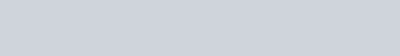The best-known of these are the arithmetic, geometric, and harmonic means, with p = 1, p = –1, and  p → 0: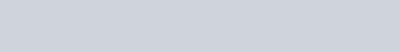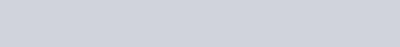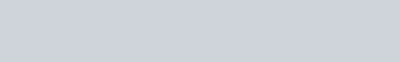The other well-known power mean is the root mean square with p = 2: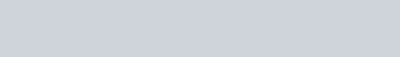These are all examples of quasi-arithmetic means: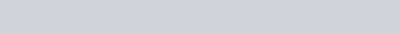The power means suggest power operations: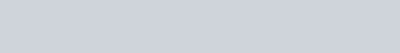These include arithmetic addition (p = 1), reciprocal addition (p = –1), and the root sum squared (p = 2):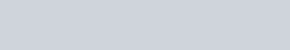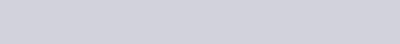In order to avoid roots of negative numbers, the absolute value is often taken: | ai |. If the ai represent differences such as | xiyi |, then the operations are p-norms: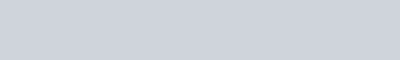The best-known norm is the Euclidean norm or distance (p = 2):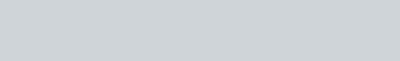Reciprocal addition is part of reciprocal arithmetic, see here.